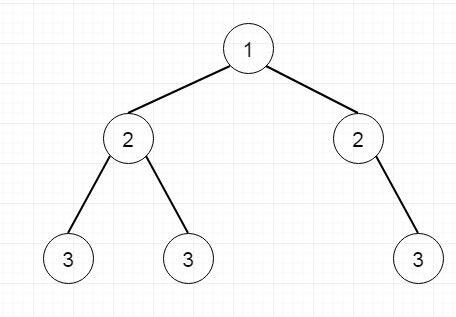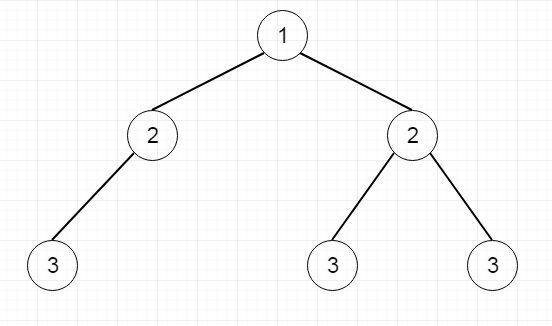# Invert Binary Tree in Python

Suppose we have a binary tree. our task is to create an inverted binary tree. So if the tree is like below −The inverted tree will be likeTo solve this, we will use a recursive approach

• if the root is null, then return
• swap the left and right pointers
• recursively solve left subtree and right subtree

## Example (Python)

Let us see the following implementation to get a better understanding −

Live Demo

class TreeNode:
def __init__(self, data, left = None, right = None):
self.data = data
self.left = left
self.right = right
def make_tree(elements):
Tree = TreeNode(elements)
for element in elements[1:]:
insert(Tree, element)
return Tree
def height(root):
if root is None:
return 0
else :
# Compute the height of left and right subtree
l_height = height(root.left)
r_height = height(root.right)
#Find the greater one, and return it
if l_height > r_height :
return l_height+1
else:
return r_height+1
def print_given_level(root, level):
if root is None:
return
if level == 1:
print(root.data,end = ',')
elif level > 1 :
print_given_level(root.left , level-1)
print_given_level(root.right , level-1)
def level_order(root):
h = height(root)
for i in range(1, h+1):
print_given_level(root, i)
def insert(temp,data):
que = []
que.append(temp)
while (len(que)):
temp = que
que.pop(0)
if (not temp.left):
if data is not None:
temp.left = TreeNode(data)
else:
temp.left = TreeNode(0)
break
else:
que.append(temp.left)
if (not temp.right):
if data is not None:
temp.right = TreeNode(data)
else:
temp.right = TreeNode(0)
break
else:
que.append(temp.right)
class Solution(object):
def invertTree(self, root):
"""
:type root: TreeNode
:rtype: TreeNode
"""
self.solve(root)
return root
def solve(self,root):
if not root:
return
temp = root.left
root.left = root.right
root.right = temp
self.solve(root.left)
self.solve(root.right)
tree1 = make_tree([1,2,2,3,4,None,3])
ob1 = Solution()
tree2 = ob1.invertTree(tree1)
level_order(tree2)

## Input

[1,2,2,3,4,None,3]

## Output

1,2,2,3,None,4,3,